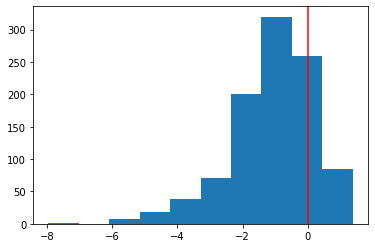# YeoJonson transformation

YeoJonson transform is one of the transform methods that bring numerical data closer to a normal distribution. Unlike the BoxCox transformation, which cannot be applied to data containing negative values, it can be applied even when negative values are included.

Unlike the box-cox transformation, this method can be used even when negative values are included.

from scipy import stats
import matplotlib.pyplot as plt

x = stats.loggamma.rvs(1, size=1000) - 0.5
plt.hist(x)
plt.axvline(x=0, color="r")  # verify that there is data below 0 as well
plt.show()import numpy as np
from scipy.stats import yeojohnson

plt.hist(yeojohnson(x))
plt.show()